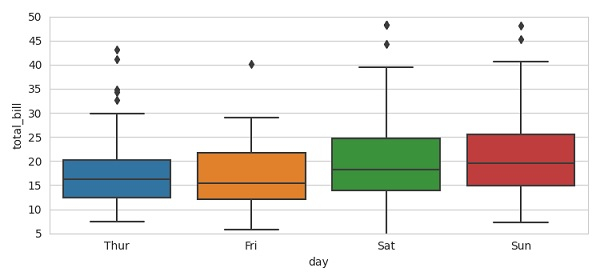# How to set the range of Y-axis for a Seaborn boxplot using Matplotlib?

To set the range of Y-axis for a Seaborn boxplot, we can take the following steps −

• Using set_style() method, set the aesthetic style of the plots.

• Using boxplot(), draw a box plot to show distributions with respect to categories.

• To set the range of Y-axis, use the ylim() method.

• To display the figure, use the show() method.

## Example

from matplotlib import pyplot as plt
import seaborn as sns
plt.rcParams["figure.figsize"] = [7.50, 3.50]
plt.rcParams["figure.autolayout"] = True
sns.set_style("whitegrid")
plt.show()